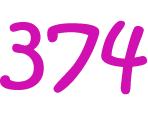# Definition of OctalOctal numbers are made up only of the digits $\{0,1,2,3,4,5,6,7\}$. Each place in an octal number corresponds to a power of $8$.

Because there are only $8$ different possible digits in an octal number, we also call them Base $8$ numbers.

For example, the octal number $374$ is equal to the decimal number $3 \times 8^2 + 4 \times 8^1 + 7 \times 8^0 = 3 \times 64 + 4 \times 8 + 7 \times 1 = 231$.

Some other examples of octal numbers are:

• $77$, which corresponds to the decimal number $63$.
• $100$, which corresponds to the decimal number $64$.
• $101$, which corresponds to the decimal number $65$.

### Description

The aim of this dictionary is to provide definitions to common mathematical terms. Students learn a new math skill every week at school, sometimes just before they start a new skill, if they want to look at what a specific term means, this is where this dictionary will become handy and a go-to guide for a student.

### Audience

Year 1 to Year 12 students

### Learning Objectives

Learn common math terms starting with letter O

Author: Subject Coach
You must be logged in as Student to ask a Question.